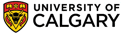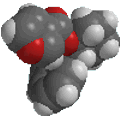Chapter 25: CarbohydratesAldoses and Ketoses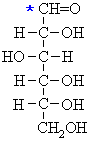or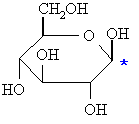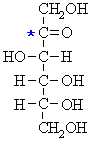or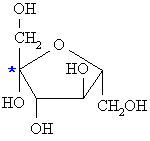D-glucose an aldose an aldohexose D-fructose a ketose  a ketohexose

Summary

• Carbohydrates are really just polyhydroxyaldehydes, the aldoses, or polyhydroxyketones, the ketoses.
• So, for a carbohydrate to be an aldose it needs an aldehyde group.
• Remember that an aldehyde group, here shown as CH=O, is also often written as CHO.
• And to be a ketose it needs a ketone group.
• The critical centers are marked in the diagrams with *
• The assignment is quite apparent in the acyclic form of the sugar, see above.
• In the cyclic form, find the anomeric center (*) and then look at the substituents there. If one is an H, then it is an aldose (see glucose example above).
• The systems are further classified depending on how many C atoms there are (see above for examples)
• 4 C = tetrose
• 5 C = pentose
• 6 C = hexose© Dr. Ian Hunt, Department of Chemistry